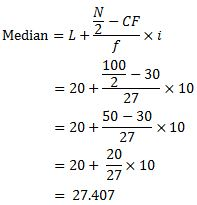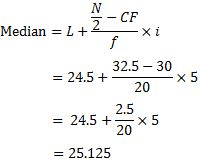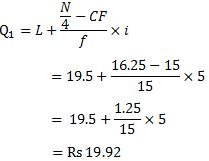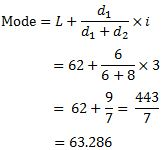#### NCERT Solutions for Class 11th: Ch 5 Measures of Central Tendency Statistics for Economics

Page No: 71

Exercises

1. Which average would be suitable in the following cases?

► Mode

(ii) Average intelligence of students in a class.
► Median

(iii) Average production in a factory per shift.
► Arithmetic average

(iv) Average wages in an industrial concern.
► Arithmetic average

(v) When the sum of absolute deviations from average is least.
► Median

(vi) When quantities of the variable are in ratios.
► Arithmetic average

(vii) In case of open-ended frequency distribution.
► Median

2. Indicate the most appropriate alternative from the multiple choices provided against each question.

(i) The most suitable average for qualitative measurement is
(a) arithmetic mean
(b) median
(c) mode
(d) geometric mean
(e) none of the above
► (b) median

Page No: 72

(ii) Which average is affected most by the presence of extreme items?
(a) median
(b) mode
(c) arithmetic mean
(d) none of the above
► (c) arithmetic mean

(iii) The algebraic sum of deviation of a set of n values from A.M. is
(a) n
(b) 0
(c) 1
(d) none of the above
► (b) 0

3. Comment whether the following statements are true or false.

(i) The sum of deviation of items from median is zero.
► False

(ii) An average alone is not enough to compare series.
► True

(iii) Arithmetic mean is a positional value.
► False

(iv) Upper quartile is the lowest value of top 25% of items.
► True

(v) Median is unduly affected by extreme observations.
► False

4. If the arithmetic mean of the data given below is 28, find (a) the missing frequency, and (b) the median of the series:

 Profit per retail shop (in Rs) 0-10 10-20 20-30 30-40 40-50 50-60 Number of retail shops 12 18 27 - 17 6

(a) Let the missing frequency be x
Arithmetic mean = 28 (given)

 Profit per retail shop (in Rs) Class Interval No. of retail shops Frequency (f) Mid Value (m) fm 0-10 12 5 60 10-20 18 15 270 20-30 27 25 675 30-40 x 35 35x 40-50 17 45 765 50-60 6 55 330 Σf = 80 + x Σfx = 2100 + 35x

Mean = Σfx/Σf
⇒ 28 = 2100 + 35x/80 + x
⇒ 2240 + 28x = 2100 + 35
⇒ 2240 - 2100 = 35x - 25x
⇒ 140 = 7x
⇒  x = 140/7 = 20
Missing frequency = 20

(b)
 Class Interval Frequency (f) Cumulative frequency (CF) 0-10 12 12 10-20 18 30 20-30 27 57 30-40 x 77 40-50 17 94 50-60 6 100 Total Σf = 100

Median = Size of (N/2)th item
= 100/2 = 50th item
It lies in class 20-30.5. The following table gives the daily income of ten workers in a factory. Find the arithmetic mean.

 Workers A B C D E F G H I J Daily Income (in Rs) 120 150 180 200 250 300 220 350 370 260

 Workers Daily Income (in Rs) X A 120 B 150 C 180 D 200 E 250 F 300 G 220 H 350 I 370 J 260 Total ΣX = 2400

N = 10
Arithmetic Mean = ΣX/N
= 2400/10
= 240
Arithmetic Mean = 240

6. Following information pertains to the daily income of 150 families. Calculate the arithmetic mean.
 Income (in Rs) Number of families More than 75 150 More than 85 140 More than 95 115 More than 105 95 More than 115 70 More than 125 60 More than 135 40 More than 145 25

 Income No. of families Frequency (f) Mid Class (x) fx 75-85 10 80 800 85-95 25 90 2250 95-105 20 100 2000 105-115 25 110 2750 115-125 10 120 1200 125-135 20 130 2600 135-145 15 140 2100 145-155 25 150 3750 150 17450
Arithmetic Mean = Σfx/Σf
= 17450/150
= 116.33

7. The size of land holdings of 380 families in a village is given below. Find the median size of land holdings.
 Size of Land Holdings (in acres) Less than 100 100-200 200-300 300-400 400 and above Number of families 40 89 148 64 39

 Size of Land Holdings        Class Interval Number of families (f) Cumulative frequency (CF) 0-100 40 40 100-200 89 129 200-300 148 277 300-400 64 341 400-500 39 380 Total Σf = 380
Σf = N = 380
Median = Size of (N/2)th item
= 380/2 = 190th item
It lies in class 200-300.Median size of land holdings = 241.22 acres

8. The following series relates to the daily income of workers employed in a firm. Compute (a) highest income of lowest 50% workers (b) minimum income earned by the top 25% workers and (c) maximum income earned by lowest 25% workers.
 Daily Income (in Rs) 10-14 15-19 20-24 25-29 30-34 35-39 Number of workers 5 10 15 20 10 5
(Hint: Compute median, lower quartile and upper quartile.)

 Daily Income (in Rs)       Class Interval No of workers (f) Cumulative frequency (CF) 9.5-14.5 5 5 14.5-19.5 10 15 19.5-24.5 15 30 24.5-29.5 20 50 29.5-34.5 10 60 34.5-39.5 5 65 Total Σf = 65

(a) Σf = N = 65
Median = Size of (N/2)th item
= 65/2 = 32.5th item
It lies in class 24.5-29.5.Highest income of lowest 50% workers = Rs 25.12

(b) First, we need to find Q1
Class interval of Q1 = (N/4)th items
= (65/4)th items = 16.25th item
It lies in class 19.5-24.5.Minimum income earned by the top 25% workers = Rs 19.92

(c) First, we need to find Q3
Class interval of Q= 3 (N/4)th items
= 3 (65/4)th items = 3 × 16.25th item
= 48.75th item
It lies in class 24.5-29.5.Maximum income earned by lowest 25% workers = Rs 29.19

9. The following table gives production yield in kg. per hectare of wheat of 150 farms in a village. Calculate the mean, median and mode values.
 Production yield (kg. per hectare) 50-53 53-56 56-59 59-62 62-65 65-68 68-71 71-74 74-77 Number of workers 3 8 14 30 36 28 16 10 5